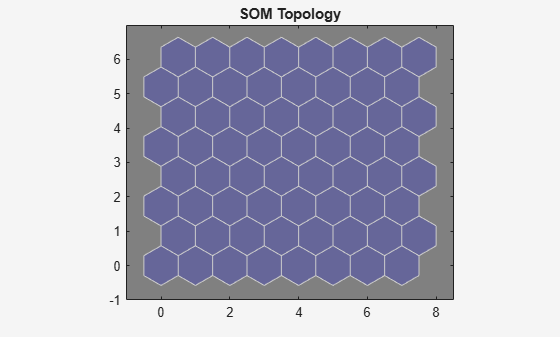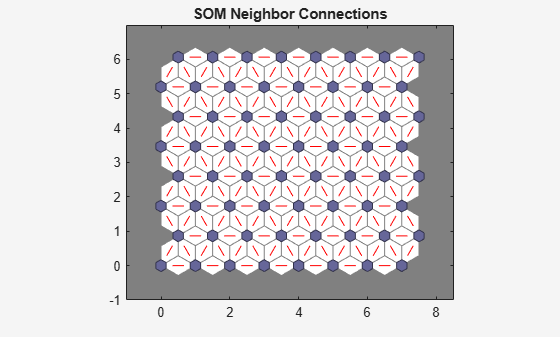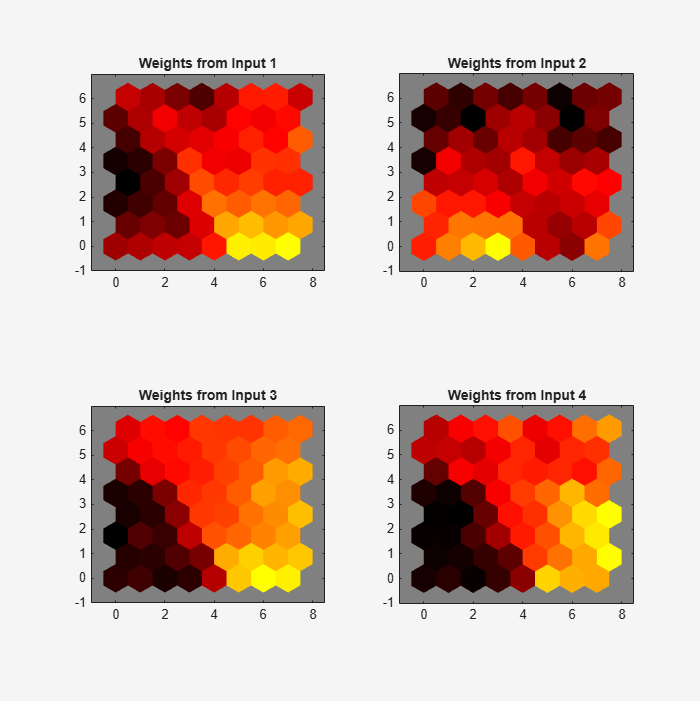Documentation

## Iris Clustering

This example illustrates how a self-organizing map neural network can cluster iris flowers into classes topologically, providing insight into the types of flowers and a useful tool for further analysis.

### The Problem: Cluster Iris Flowers

In this example we attempt to build a neural network that clusters iris flowers into natural classes, such that similar classes are grouped together. Each iris is described by four features:

• Sepal length in cm

• Sepal width in cm

• Petal length in cm

• Petal width in cm

This is an example of a clustering problem, where we would like to group samples into classes based on the similarity between samples. We would like to create a neural network which not only creates class definitions for the known inputs, but will let us classify unknown inputs accordingly.

### Why Self-Organizing Map Neural Networks?

Self-organizing maps (SOMs) are very good at creating classifications. Further, the classifications retain topological information about which classes are most similar to others. Self-organizing maps can be created with any desired level of detail. They are particularly well suited for clustering data in many dimensions and with complexly shaped and connected feature spaces. They are well suited to cluster iris flowers.

The four flower attributes will act as inputs to the SOM, which will map them onto a 2-dimensional layer of neurons.

### Preparing the Data

Data for clustering problems are set up for a SOM by organizing the data into an input matrix X.

Each ith column of the input matrix will have four elements representing the four measurements taken on a single flower.

Here such a dataset is loaded.

```x = iris_dataset; ```

We can view the size of inputs X.

Note that X has 150 columns. These represent 150 sets of iris flower attributes. It has four rows, for the four measurements.

```size(x) ```
```ans = 4 150 ```

### Clustering with a Neural Network

The next step is to create a neural network that will learn to cluster.

selforgmap creates self-organizing maps for classifying samples with as much detail as desired by selecting the number of neurons in each dimension of the layer.

We will try a 2-dimension layer of 64 neurons arranged in an 8x8 hexagonal grid for this example. In general, greater detail is achieved with more neurons, and more dimensions allows for modelling the topology of more complex feature spaces.

The input size is 0 because the network has not yet been configured to match our input data. This will happen when the network is trained.

```net = selforgmap([8 8]); view(net) ```Now the network is ready to be optimized with train.

The Neural Network Training Tool shows the network being trained and the algorithms used to train it. It also displays the training state during training and the criteria which stopped training will be highlighted in green.

The buttons at the bottom open useful plots which can be opened during and after training. Links next to the algorithm names and plot buttons open documentation on those subjects.

```[net,tr] = train(net,x); nntraintool ```Here the self-organizing map is used to compute the class vectors of each of the training inputs. These classifications cover the feature space populated by the known flowers, and can now be used to classify new flowers accordingly. The network output will be a 64x150 matrix, where each ith column represents the jth cluster for each ith input vector with a 1 in its jth element.

The function vec2ind returns the index of the neuron with an output of 1, for each vector. The indices will range between 1 and 64 for the 64 clusters represented by the 64 neurons.

```y = net(x); cluster_index = vec2ind(y); ```

plotsomtop plots the self-organizing maps topology of 64 neurons positioned in an 8x8 hexagonal grid. Each neuron has learned to represent a different class of flower, with adjacent neurons typically representing similar classes.

```plotsomtop(net) ```plotsomhits calculates the classes for each flower and shows the number of flowers in each class. Areas of neurons with large numbers of hits indicate classes representing similar highly populated regions of the feature space. Whereas areas with few hits indicate sparsely populated regions of the feature space.

```plotsomhits(net,x) ```plotsomnc shows the neuron neighbor connections. Neighbors typically classify similar samples.

```plotsomnc(net) ```plotsomnd shows how distant (in terms of Euclidian distance) each neuron's class is from its neighbors. Connections which are bright indicate highly connected areas of the input space. While dark connections indicate classes representing regions of the feature space which are far apart, with few or no flowers between them.

Long borders of dark connections separating large regions of the input space indicate that the classes on either side of the border represent flowers with very different features.

```plotsomnd(net) ```plotsomplanes shows a weight plane for each of the four input features. They are visualizations of the weights that connect each input to each of the 64 neurons in the 8x8 hexagonal grid. Darker colors represent larger weights. If two inputs have similar weight planes (their color gradients may be the same or in reverse) it indicates they are highly correlated.

```plotsomplanes(net) ```This example illustrated how to design a neural network that clusters iris flowers based on four of their characteristics.

Explore other examples and the documentation for more insight into neural networks and their applications.

Download ebook